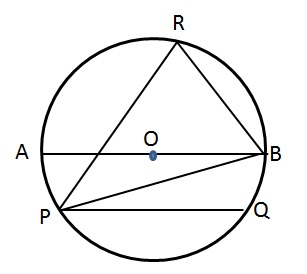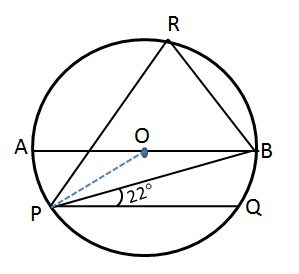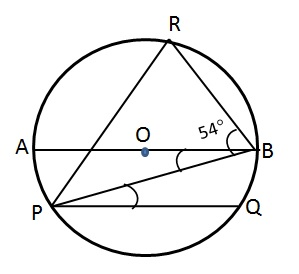# CAT Quantitative Aptitude Questions | Geometry Questions for CAT - Triangles

###### CAT Questions | CAT Geometry Questions | Circles

The question is from the topic circles. It is a data sufficiency question. Finding the angle subtended by a chord at the centre would help. CAT Geometry questions are heavily tested in CAT exam. Make sure you master Geometry problems.

Question 32: Find ∠PRB. Given
I. ∠BPQ = 22 and O is the centre of the circle
II. ∠RBP = 54 and chord PQ is parallel to AB1. Either I or II individually is sufficient
2. Both I and II together are required
3. One of the statements alone is sufficient
4. Need more data

## Best CAT Coaching in Chennai

#### CAT Coaching in Chennai - CAT 2021Online Batches Available Now!

##### Method of solving this CAT Question from Circles: Try to find the angle subtended by the chord PB at the center from the given statements!

Statement I
O is the centre implies AB is the diameter
∠BPQ = 22
Let us join OP and see what we get.OP = OB
∠OPB = ∠OBP (Triangle OPB is isosceles)
If only we know ∠POB, then we can find the answer…

Statement II
∠RBP = 54Chord PQ is parallel to AB implies ∠BPQ = ∠ABP
We definitely need one of the above angle to get some clue…
Let us combine both the statements
Since ∠BPQ = 22, ∠ABP = 22
∠POB = 180 – (22 + 22) = 136
∠PRB is nothing but the angle subtended by the chord PB and is half the angle at the center.
∠PRB = ∠POB / 2 = 136/ 2 = 68
We need both statements together to arrive at a solution!

The question is "Choose the correct answer"

##### Hence, the answer is Both I and II together are required.

Choice B is the correct answer.

###### CAT Coaching in ChennaiCAT 2021Enroll at 49,000/- 44,000/-

Online Classroom Batches Starting Now!

###### Best CAT Coaching in ChennaiPrices slashed by Rs 4000/-

Attend a Demo Class

## CAT Preparation Online | CAT Geometry questions Videos On YouTube

#### Other useful sources for Geometry Question | Geometry Triangles Circles Quadrilaterals Sample Questions

##### Where is 2IIM located?

2IIM Online CAT Coaching
A Fermat Education Initiative,
58/16, Indira Gandhi Street,
Kaveri Rangan Nagar, Saligramam, Chennai 600 093

##### How to reach 2IIM?

Phone: (91) 44 4505 8484
Mobile: (91) 99626 48484
WhatsApp: WhatsApp Now
Email: prep@2iim.com